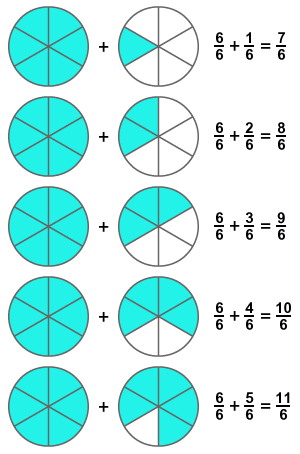# Improper Fraction

Definition:
A fraction which contains the number of selected parts of a whole is greater than the total number of parts of the same whole, is called improper fraction.

A whole quantity can be divided into numerous equal parts and the selected portion from them is known as fraction. The number of selected parts from them should always be less than the total number of parts. It is improper if the number of selected parts is greater than the total number of parts. Hence, the fraction is called improper fraction.

The values of such fractions are always greater than 1 due to the ratio of greater selected number of parts to total number of parts of the whole quantity. Actually, the selected number of parts are greater if parts of another whole quantity are involved in forming the fraction.

## Example

Consider two circles and divide each circle into six sectors.1. Consider six parts from one circle and one part of another circle. The fraction of them is $\frac{7}{6}$
2. Consider six parts from one circle and two parts of second circle. The fraction of them is $\frac{8}{6}$
3. Consider six parts from first circle and three parts from the remaining circle. The fraction of them is $\frac{9}{6}$
4. Consider six parts from first circle and four parts from the second circle. The fraction of them is $\frac{10}{6}$
5. Consider six parts from the first circle and five parts from the second circle. The fraction of them is $\frac{11}{6}$

Six parts cannot be considered from second circle because it forms full circle.

The fractions $\frac{7}{6}$, $\frac{8}{6}$, $\frac{9}{6}$, $\frac{10}{6}$ and $\frac{11}{6}$ are five rational numbers. All of them are having same denominator but numerators are different. The numerators are greater than denominators. Actually, the total number of parts are 12 but six parts are commonly formed the fraction. It is improper. So, the fraction is called improper fraction.

Calculate the value of each fraction numerically.

The value of every fraction exceeds 1. Therefore, it is proved that the value of every improper fraction is always greater than 1.

Latest Math Topics
Latest Math Problems
Email subscription
Math Doubts is a best place to learn mathematics and from basics to advanced scientific level for students, teachers and researchers. Know more
Follow us on Social Media
###### Math Problems

Learn how to solve easy to difficult mathematics problems of all topics in various methods with step by step process and also maths questions for practising.

Learn more